Start typing, then use the up and down arrows to select an option from the list.# Weak Base Strong Acid Titrations 4

Jules Bruno
148views
2
at the equivalents point, we now have an equal amount of weak base and strong acid. At the equivalence point, they're always gonna have the same moles. Let's assume here that are weak acid is zero. Initially again, we ignore the fourth species at the equivalence point. Both my week, based on strong acid completely destroy each other. So at the end, they're both zero. Do the law of conservation of Mass. We're gonna have this amount being added to our product. So at the end of this, all we have left is weak acid. Now, remember, with a weak acid or weak base in order to determine Ph, we need to use a nice chart. So we would take the most left of our weak acid divided by the total leaders used in order. Determine the concentration because we're dealing with a weak acid would use the K of that weak acid, help us isolate X, and from there we determine what our concentration of H 30 plus would be. And from there we determine what our PH value would be. So remember, when it comes to an acid based filtration, we have to keep in mind the three places in terms of our titrate shin is that before the equivalents point after the equivalence point or at the equivalence point, those three places entail using different techniques to figure out what our final Ph would be.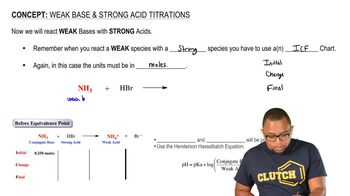01:49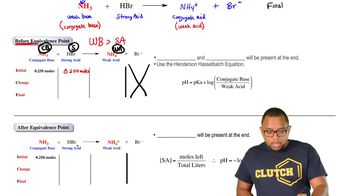02:15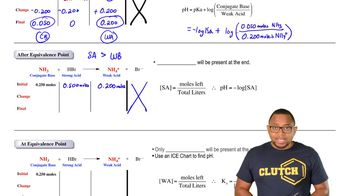01:39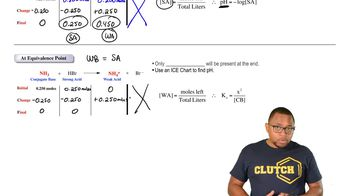01:41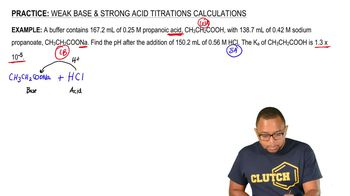05:37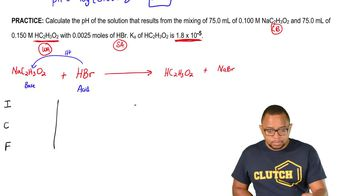04:19